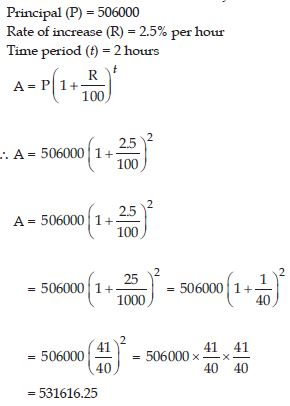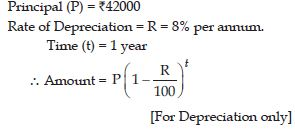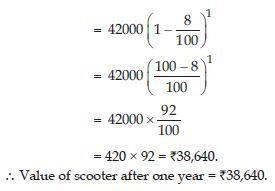# NCERT Solutions for Class 8 Math Chapter 8 - Comparing Quantities

##### Question 1: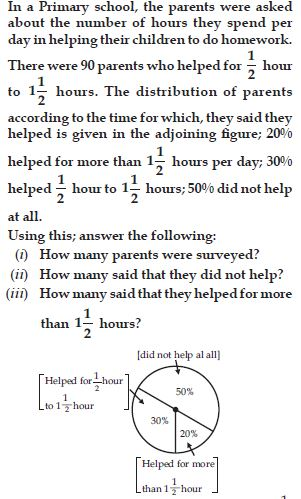##### Question 2:

Find the ratio of the following. Speed of a cycle 15 km per hour to the speed of scooter which is 30 km per hour.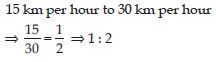##### Question 3:

Find the ratio of the following.
5 m to 10 km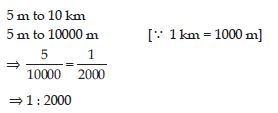##### Question 4:

Find the ratio of the following.
50 paise to ₹5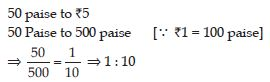##### Question 5:

Convert the following ratio to percentage:
3 : 4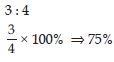##### Question 6:

Convert the following ratio to percentage:
2 : 3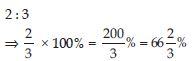##### Question 7:

72% of 25 students are good in mathematics. How many are not good in mathematics?

72% of total students are good in mathematics.
∴ 100 – 72 = 28% of total students are not good in mathematics.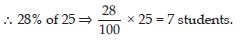∴ 7 students are not good in mathematics.

##### Question 8:

A football team won 10 matches out of the total number of matches they played. If their win percentage was 40, then how many matches did they play in all?

Let total number of matches played = x
Matches won = 10 games
Percentage won = 40% of x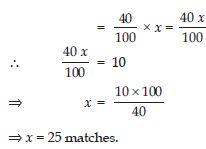##### Question 9:

If Chameli had ₹600 left after spending 75% of her money, how much did she have in the beginning?

Let the total money Chameli had = ₹x
Money left after spending = ₹600
Percentage of money spent = 75%
∴ Percentage of money left after spending
= 100 – 75 = 25%
∴ 25% of x = 600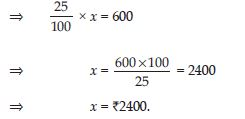##### Question 10:

If 60% people in a city like cricket, 30% like football and remaining like other games, then what per cent of the people like other games?
If the total number of people are 50 lakh, find the exact number who like each type of game.

Total number of people = 50 lakh
Percentage of people who like cricket = 60%
Percentage of people who like football = 30%
Percentage of people who like other games
= 100 – (60 + 30)
= 100 – 90 = 10%
∴ Number of people who like cricket
= 60% of 50 lakh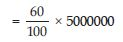= 3000000 people.
Number of people who like football
= 30% of 50 lakh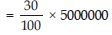= 1500000 people.
Number of people who like other games
= 10% of 50 lakh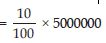= 500000 people.

##### Question 11:

A shop gives 20% discount. What would the sale price of each of these be?
(a) A dress marked at ₹120.
(b) A pair of shoes marked at ₹750.
(c) A bag marked at ₹250.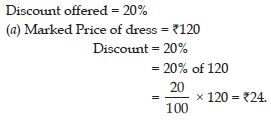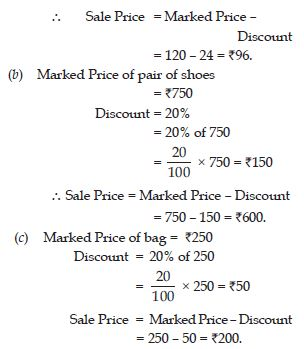##### Question 12:

A table marked at ₹15,000 is available for ₹14,400. Find the discount given and the discount per cent.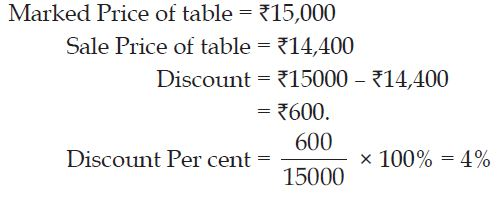##### Question 13:

An almirah is sold at ₹5225 after allowing a discount of 5%. Find its marked price.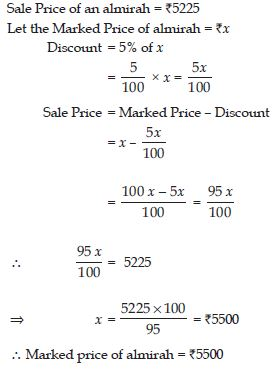##### Question 14:

Find selling price (SP) if a profit of 5% is made on:
(a) a cycle of ₹700 with ₹50 as overhead charges.
(b) a lawn mower bought at ₹1150 with ₹50 as transportation charges.
(c) a fan bought for ₹560 and expenses of ₹40 made on its repairs.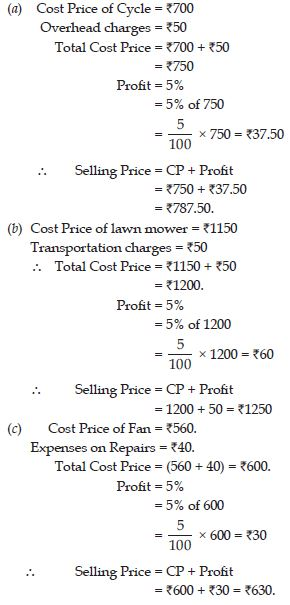##### Question 15:

A shopkeeper bought two TV sets at ₹10,000 each. He sold one at a profit of 10% and the other at a loss of 10%. Find whether he made an overall profit or loss.

Cost Price of each TV set = ₹10,000.
One is sold at a profit of 10%.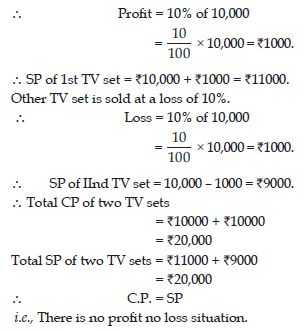##### Question 16:

Two times a number is a 100% increase in the number. If we take half the number, what would be the decrease in per cent?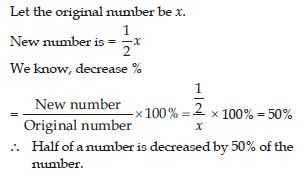##### Question 17:

By what per cent is ₹2,000 less than ₹2,400? Is it the same as the per cent by which ₹2,400 is more than ₹2,000?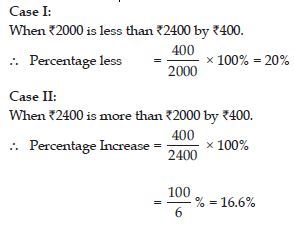##### Question 18:

A man got a 10% increase in his salary. If his new salary is ₹1,54,000, find his original salary.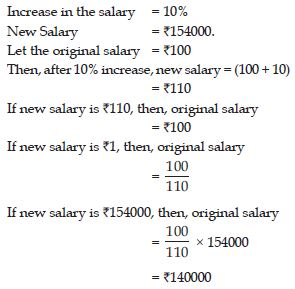##### Question 19:

On Sunday, 845 people went to the Zoo. On Monday, only 169 people went. What is the per cent decrease in the people visiting the Zoo on Monday?

Number of people went to Zoo on Sunday = 845
Number of people went to Zoo on Monday = 169
Decrease in the number of people = (845 – 169) = 676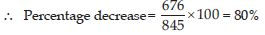##### Question 20:

A shopkeeper buys 80 articles for ₹2,400 and sells them for a profit of 16%. Find the selling price of one article.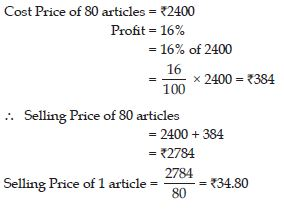##### Question 21:

The cost of an article is ₹15,500. ₹450 were spent on its repairs. If it is sold for a profit of 15%, find the selling price of the article.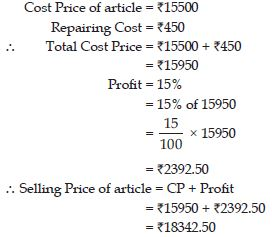##### Question 22:

A VCR and TV were bought for ₹8,000 each.
The shopkeeper made a loss of 4% on the
VCR and a profit of 8% on the TV. Find the
gain or loss per cent on the whole transaction.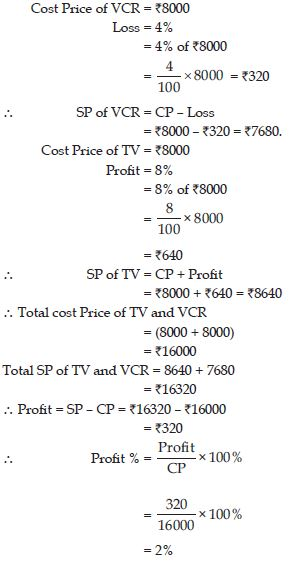##### Question 23:

During a sale, a shop offered a discount of 10% on the marked prices of all the items.
What would a customer have to pay for a pair of jeans marked at ₹1450 and two shirts marked at ₹850 each?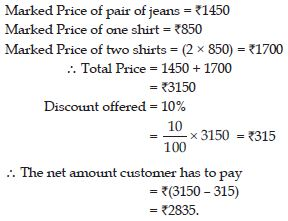##### Question 24:

A milkman sold two of his buffaloes for ₹20,000 each. On one he made a gain of 5% and on the other a loss of 10%. Find his overall gain or loss.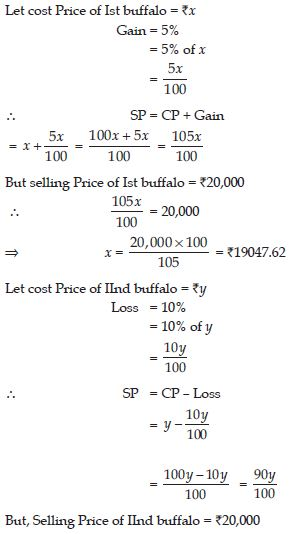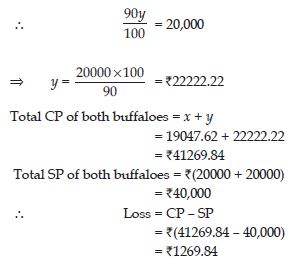##### Question 25:

The Price of a TV is ₹13,000. The sales tax charged on it is at the rate of 12%. Find the amount that Vinod will have to pay if he buys it.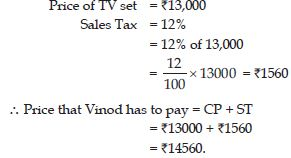##### Question 26:

Arun bought a pair of skates at a sale where the discount given was 20%. If the amount he pays is ₹1,600, find the marked price.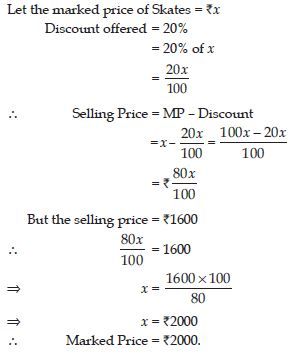##### Question 27:

I purchased a hair-dryer for ₹5,400 including 8% VAT. Find the price before VAT was added.##### Question 28:

An article was purchased for ₹ 1239 including GST of 18%. Find the price of the article before GST was added?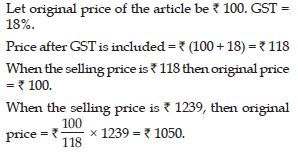##### Question 29:

Find interest and amount to be paid on ₹15000 at 5% per annum after 2 years.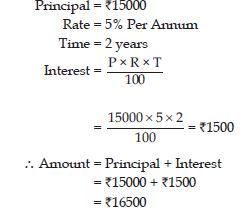##### Question 30:

Find CI on a sum of ₹8000 for 2 years at 5% per annum compounded annually.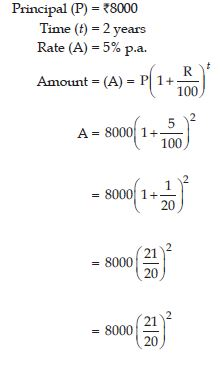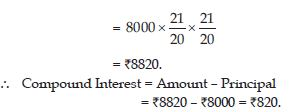##### Question 31:

Find the time period and rate for each: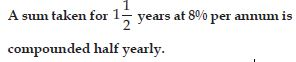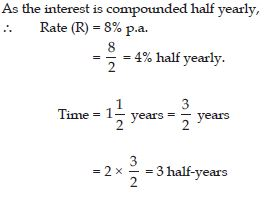##### Question 32:

A sum taken for 2 years at 4% per annum is compounded half yearly.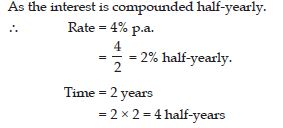##### Question 33:

A sum is taken for one year at 16% p.a. If interest is compounded after every three months, how many times will interest be charged in one year?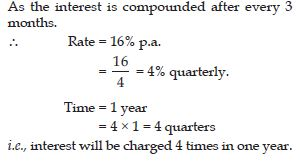##### Question 34:

Find the amount to be paid at the end of 2 years on ₹2,400 at 5% per annum compounded annually.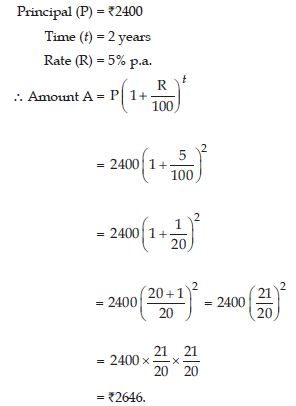##### Question 35:

At the end of 1 year on ₹1,800 at 8% per annum compounded quarterly.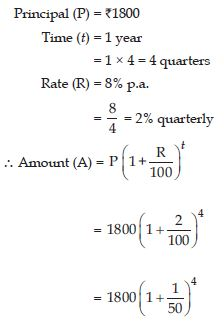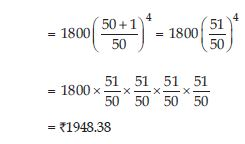##### Question 36:

A machinery worth ₹10,500 depreciated by 5%. Find its value after one year.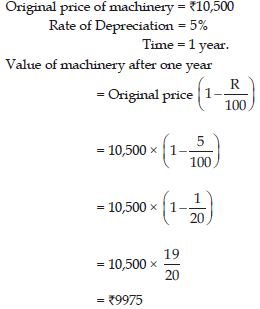##### Question 37:

Find population of a city after 2 years, which is at present 12 lakh, if the rate of increase is 4%.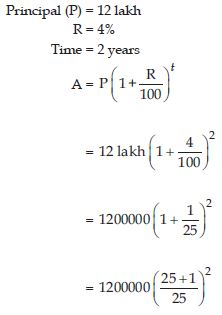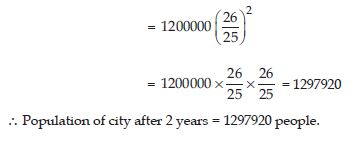##### Question 38:

Calculate the amount and compound interest on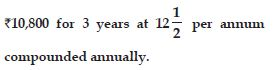Amount = ₹15377.34
∴ Compound Interest = Amount – Principal
= ₹15377.34 – ₹10800
= ₹4577.34

##### Question 39:

Calculate the amount and compound interest on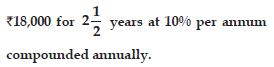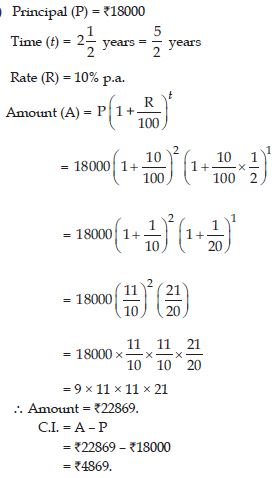##### Question 40:

Calculate the amount and compound interest on##### Question 41:

Calculate the amount and compound interest on ₹8,000 for 1 year at 9% per annum compounded half-yearly.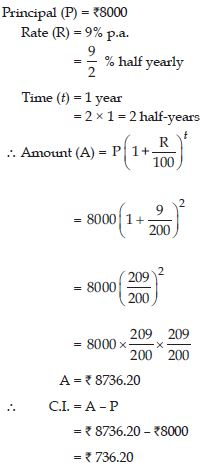##### Question 42:

Calculate the amount and compound interest on ₹10,000 for 1 year at 8% per annum compounded half-yearly.##### Question 43:

Kamla borrowed ₹26,400 from a bank to buy a scooter at a rate of 15% p.a. compounded yearly. What amount will she pay at the end of 2 years and 4 months to clear the loan?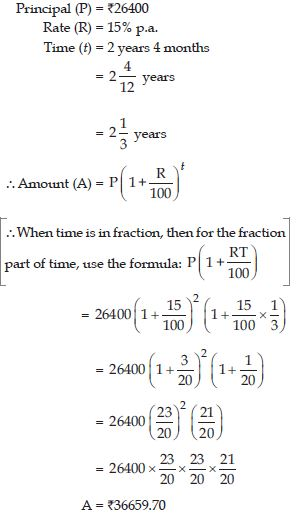##### Question 44:

Fabina borrows ₹12,500 at 12% per annum for 3 years at simple interest and Radha borrows the same amount for the same time period at 10% per annum, compounded annually. Who pays more interest and by how much?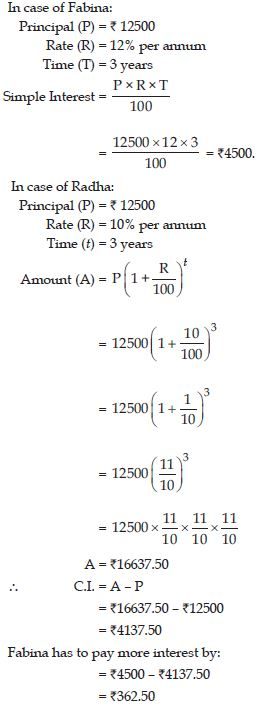##### Question 45:

I borrowed ₹12,000 from Jamshed at 6% per annum simple interest for 2 years. Had I borrowed this sum by 6% per annum compound interest, what extra amount would I have to pay?##### Question 46:

Vasudevan invested ₹60,000 at an interest rate of 12% per annum compounded half yearly. What amount would he get after 6 months?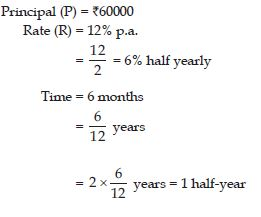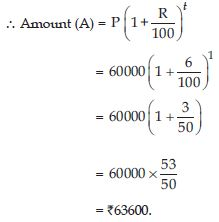##### Question 47:

Vasudevan invested ₹60,000 at an interest rate of 12% per annum compounded half yearly. What amount would he get after 1 year?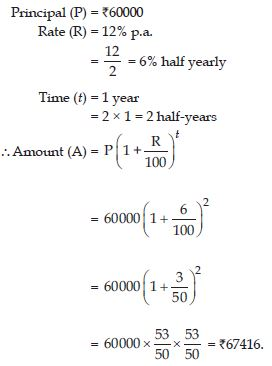##### Question 48: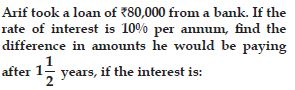(i) compounded annually
(ii) compounded half yearly.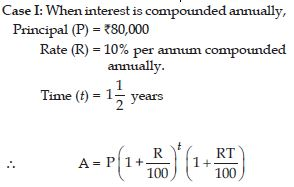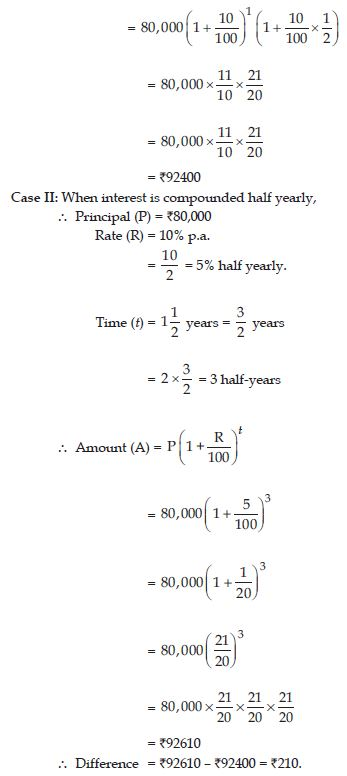##### Question 49:

Maria invested ₹8,000 in a business. She would be paid interest at 5% per annum compounded annually. Find
(i) The amount credited against her name at the end of the second year.

(ii) The interest for the 3rd year.##### Question 50: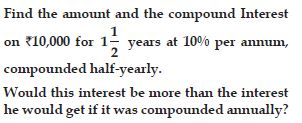Amount = ₹11576.25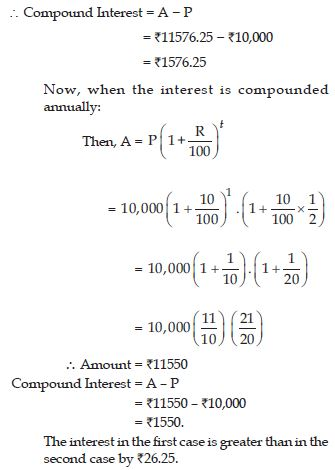##### Question 51: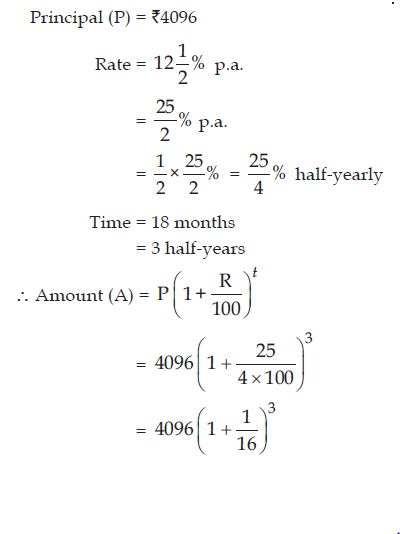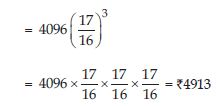##### Question 52:

The population of a place increased to 54,000 in 2003 at a rate of 5% per annum:
(i) find the population in 2001.
(ii) what would be its population in 2005?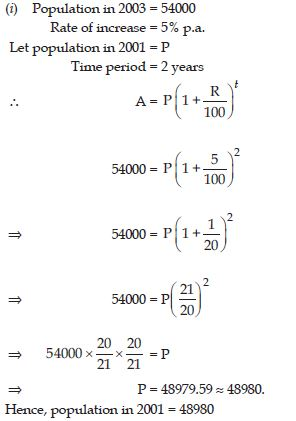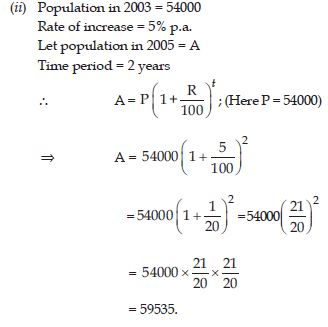##### Question 53:

In a laboratory, the count of bacteria in a certain experiment was increasing at the rate of 2.5% per hour. Find the bacteria at the end of 2 hours, if the count was initially 5,06,000.